SLUS772G MARCH   2008  – June 2020

PRODUCTION DATA.

1. Features
2. Applications
3. Description
1.     Device Images
4. Revision History
5. Pin Configuration and Functions
6. Specifications
7. Detailed Description
1. 7.1 Overview
2. 7.2 Functional Block Diagram
3. 7.3 Feature Description
4. 7.4 Device Functional Modes
8. Application and Implementation
1. 8.1 Application Information
2. 8.2 Typical Applications
1. 8.2.1 12-V to 24-V Nonsynchronous Boost Regulator
1. 8.2.1.1 Design Requirements
2. 8.2.1.2 Detailed Design Procedure
3. 8.2.1.3 Application Curves
2. 8.2.2 12-V Input, 700-mA LED Driver, Up to 35-V LED String
9. Power Supply Recommendations
10. 10Layout
11. 11Device and Documentation Support
1. 11.1 Device Support
2. 11.2 Documentation Support
6. 11.6 Electrostatic Discharge Caution
7. 11.7 Glossary
12. 12Mechanical, Packaging, and Orderable Information

• DGQ|10
• DRC|10
• DGQ|10
• DRC|10

7.3.10 Control Loop Considerations

There are two methods to design a suitable control loop for the TPS4021x. The first and preferred if equipment is available is to use a frequency response analyzer to measure the open loop modulator and power stage gain and to then design compensation to fit that. The usage of these tools for this purpose is well documented with the literature that accompanies the tool and is not be discussed here.

The second option is to make an initial guess at compensation, and then evaluate the transient response of the system to see if the compensation is acceptable to the application or not. For most systems, an adequate response can be obtained by simply placing a series resistor and capacitor (RFB and CFB) from the COMP pin to the FB pin as shown in Figure 27. The initial compensation selection can be done more accurately with aid of WEBENCH® to select the components or the average Spice model to simulate the open loop modulator and power stage gain.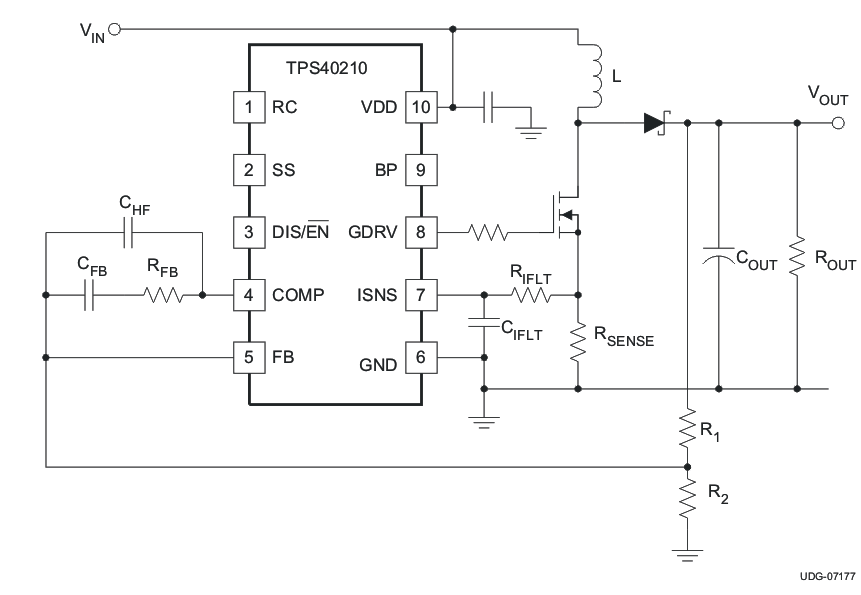Figure 27. Basic Compensation Network

The natural phase characteristics of most capacitors used for boost outputs combined with the current mode control provide adequate phase margin when using this type of compensation. To determine an initial starting point for the compensation, the desired crossover frequency must be considered when estimating the control to output gain. The model used is a current source into the output capacitor and load.

When using these equations, the loop bandwidth should be no more than 20% of the switching frequency, fSW. A more reasonable loop bandwidth would be 10% of the switching frequency. Be sure to evaluate the transient response of the converter over the expected load range to ensure acceptable operation.

Equation 22.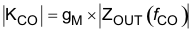Equation 23.Equation 24.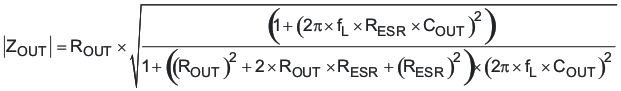where

• KCO is the control to output gain of the converter, in V/V
• gM is the transconductance of the power stage and modulator, in S
• ROUT is the output load equivalent resistance, in Ω
• ZOUT is the output impedance, including the output capacitor, in Ω
• RISNS is the value of the current sense resistor, in Ω
• L is the value of the inductor, in H
• COUT is the value of the output capacitance, in F
• RESR is the equivalent series resistance of COUT, in Ω
• f SW is the switching frequency, in Hz
• f L is the desired crossover frequency for the control loop, in Hz

These equations assume that the operation is discontinuous and that the load is purely resistive. The gain in continuous conduction can be found by evaluating Equation 23 at the resistance that gives the critical conduction current for the converter. Loads that are more like current sources give slightly higher gains than predicted here. To find the gain of the compensation network required for a control loop of bandwidth fL, take the reciprocal of Equation 22.

Equation 25.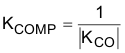The GBWP of the error amplifier is only guaranteed to be at least 1.5MHz. If KCOMP multiplied by fL is greater than 750 kHz, reduce the desired loop crossover frequency until this condition is satisfied. This ensures that the high-frequency pole from the error amplifier response with the compensation network in place does not cause excessive phase lag at fL and decreased phase margin in the loop.

The RC network connected from COMP to FB places a zero in the compensation response. That zero should be approximately 1/10th of the desired crossover frequency, fL. With that being the case, RFB and CFB can be found from Equation 26 and Equation 27.

Equation 26.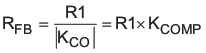Equation 27.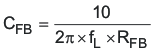where

• R1 is the high side feedback resistor in Figure 27, in Ω
• fL is the desired loop crossover frequency, in Hz

Thought not strictly necessary, it is recommended that a capacitor be added between COMP and FB to provide high-frequency noise attenuation in the control loop circuit. This capacitor introduces another pole in the compensation response. The allowable location of that pole frequency determines the capacitor value. As a starting point, the pole frequency should be 10 × fL. The value of CHF can be found from Equation 28.

Equation 28.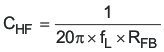While the error amplifier GBWP will usually be higher, it can be as low as 1.5MHz. If 10 × KComp × fL > 1.5MHz, the error amplifier gain-bandwidth product may limit the high-frequency response below that of the high-frequency capacitor. To maintain a consistent high-frequency gain roll-off, CHF can be calculated by Equation 29.

Equation 29.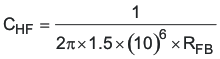where

• CHF is the high-frequency roll-off capacitor value in F
• RFB is the mid band gain setting resistor value in Ω